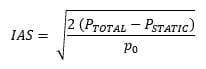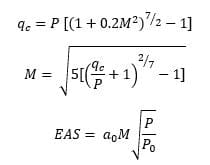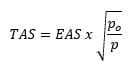Ask a racecar driver what their top speed is, and it is an easy answer. No calculations required. The same applies to a sprinter or marathon runner. Airspeed in the world of aviation, however, is a more complex topic. We have not just one type of airspeed, but many, and each has a different purpose.

Today we will focus on true airspeed including a review of what true airspeed is, how to calculate true airspeed, and the purpose of true airspeed.

## What is true airspeed?

The FAA defines true airspeed or TAS as “the airspeed of an aircraft relative to undisturbed air True airspeed is a calculated airspeed which has been adjusted for both altitude and non-standard air. True airspeed is used for navigational and aircraft performance purposes.

## How do you measure and calculate TAS using a rule of thumb?

The rule of thumb method is the least precise, but fastest and easiest way to estimate true airspeed. The general rule of thumb is that true airspeed is an additional roughly 2% higher than indicated airspeed for each 1,000 feet above sea level.

## How do you measure and calculate TAS using a flight app?

The easiest way to accurately calculate TAS is to have a digital device app do it for you. All you need to do is gather the data and input it so your app can handle all the behind-the-scenes computations. Follow the below steps to calculate true airspeed using a flight app:

### · Input variables into app

Once all the information is entered into the app, it will generate a true airspeed value for you with no math involved on your part.

## How do you measure and calculate TAS manually?

The final method of determining your true airspeed is to do a series of calculations manually using other known variables.

When learning how to manually calculate true airspeed (TAS), remember that TAS cannot be directly measured. It is calculated using other variables including outside air temperature (OAT), pressure altitude, and indicated airspeed (IAS) depending on which calculation method you use.

For those who want to understand more about the actual math that goes on behind the scenes of the flight computer apps, break out your E6B calculator, and follow the below steps to learn how to calculate the true airspeed:

### Measure indicated airspeed

Indicated airspeed is measured using the pitot-static system. Indicated airspeed is based on the measured air pressure difference between static and dynamic pressures outside the aircraft. This reading is converted to airspeed and displaced on the airspeed indicator gauge in the cockpit.

Although the airspeed indicator gauge does the calculations for us, the equation for indicated airspeed is:In the above equation,  represents the density of air in standard atmosphere.  includes both static and dynamic pressure values, while  is only the value of the static pressure reading.

There is an increasing difference between the indicated and true airspeeds as you gain altitude because the pitot tube which provides data for the airspeed indicator is measuring pressure not speed, and pressure decreases with altitude.  Thus, holding a consistent indicated airspeed while climbing will mean that the true airspeed will need to increase. Likewise holding a consistent true airspeed in a climb will result in a decreasing indicated airspeed reading.

For these reasons, we cannot simply use the indicated airspeed (IAS) reading on our gauge as a substitute for true airspeed. We must modify the IAS based on known variables to arrive at our true airspeed.

### Convert indicated airspeed into calibrated airspeed

Once you have the indicated airspeed reading, you must convert indicated airspeed into calibrated airspeed. This corrects for instrument and positional errors generated by the nature of the pitot-static system.

Read the indicated airspeed from the airspeed indicator gauge, then consult your aircraft-specific calibration table found in Section 5 of the pilot operating handbook (POH) to determine the corresponding calibrated airspeed.

### Calculate equivalent airspeed (if applicable)

Next, the textbooks will say to convert the calibrated airspeed to the equivalent airspeed. This step corrects for compressibility effects and is required if you are flying at speeds above 200 knots CAS and at altitudes above 10,000’.

At slower speeds and lower altitudes, the difference between calibrated airspeed and equivalent airspeed is negligible. If this is the case, you may skip to the next step and use the calibrated airspeed obtained from the previous step as a substitution for the equivalent airspeed.

For pilots who need to calculate equivalent airspeed, it can be done by first using other variables to calculate impact pressure which accounts for the compressibility effects. We will need to know the impact pressure and static pressure to begin. These variables will then be used to determine Mach number and subsequently our equivalent airspeed.

The series of equations to arrive at our equivalent airspeed in this manner are as follows:In the above equations,  represents the impact pressure, M is the Mach number, P is the static pressure,  is the static sea level pressure (ISA), and  is the sonic speed at sea level (ISA)

### Use equivalent airspeed to calculate true airspeed

Finally, now that you have gathered all of the variables necessary, use the following equation to determine the true airspeed by modifying the equivalent airspeed for temperature and pressure altitude variables:In the above equation, TAS is the true airspeed, EAS represents the equivalent airspeed,  is the sea level air density, and  is the actual air density at current altitude.

Remember that if you are flying below 10,000’ and at speeds of 200 knots CAS or less, you can substitute your calibrated airspeed for the equivalent airspeed in this equation.

## Why do you calculate true airspeed?

Now that we’ve made it through the mind-bending pilot math, or allowed technology to do it for us, what is the purpose of calculating true airspeed? True airspeed is primarily used for flight planning purposes and in aircraft performance specifications. It is the type of airspeed that the performance charts in your POH are based on and it is used when navigating by pilotage or dead reckoning.

When planning a flight, your FAA flight plan form will ask for true airspeed. This airspeed is especially useful for longer cross-country flight planning since, if you base your fuel burn and flight time estimates on indicated airspeed rather than true airspeed, your estimates are likely to be significantly off at higher altitudes and faster speeds. Taking the time to calculate true airspeed, by whichever means you choose, allows you to plan your flight more accurately and effectively.

AircraftEducationTraining